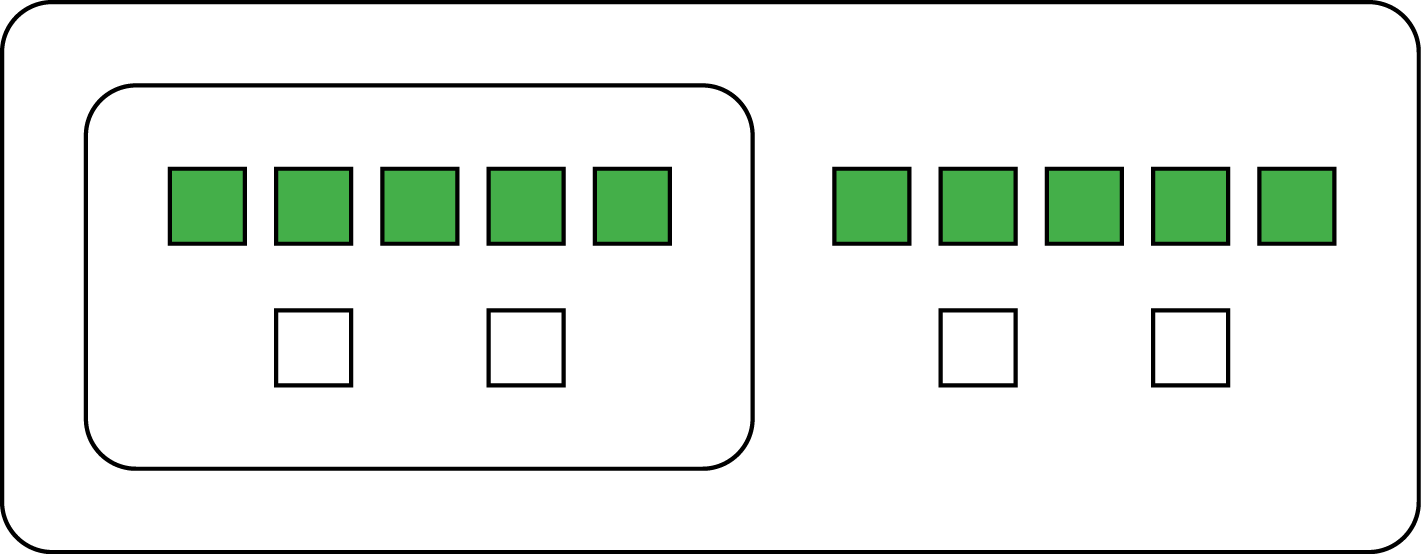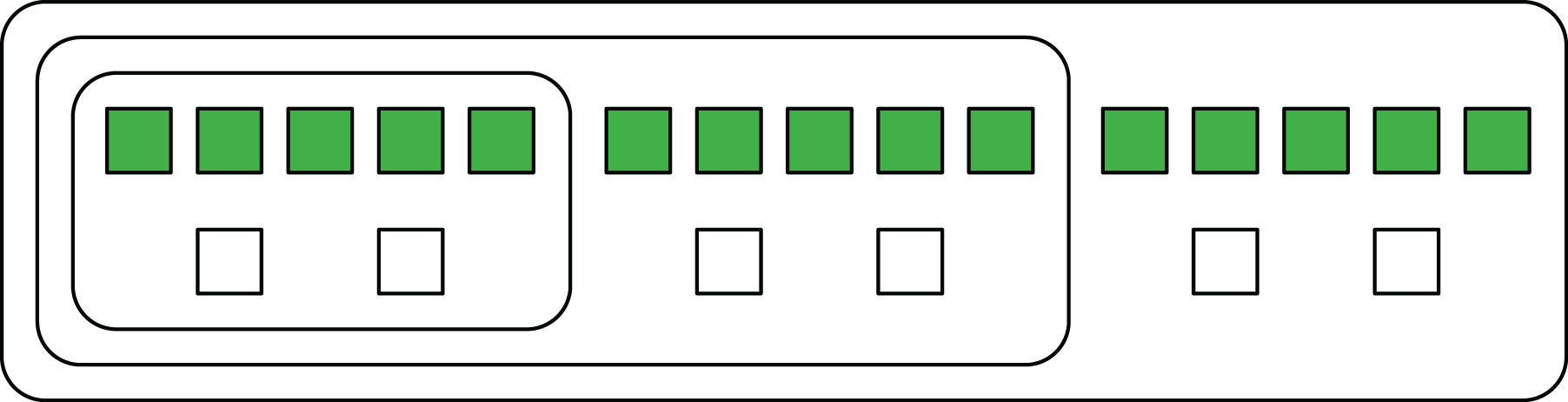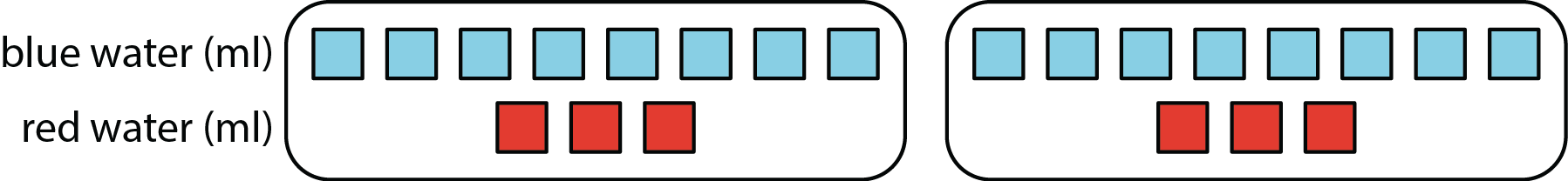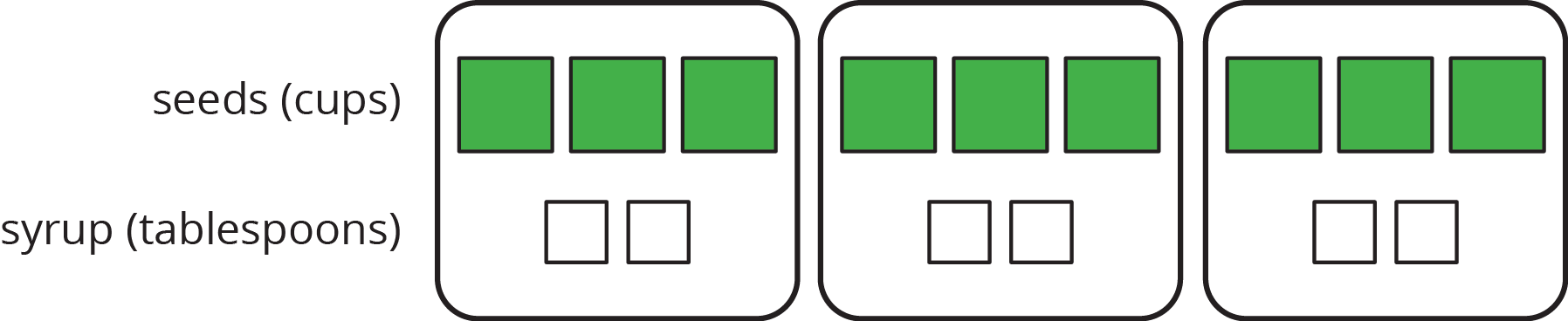Author:
Angela Vanderbloom
Subject:
Mathematics
Material Type:
Lesson Plan
Level:
Middle School
6
Tags:
• 6.RP.A.3a Equivalent Ratios
Language:
English

# 6.RP.3a Lesson 1

## Overview

This is the first of two lessons that develop the idea of equivalent ratios.

# Warm Up: Flower Pattern

The purpose of this warm-up is to quickly remind students of different ways to write ratios. They also have an opportunity to multiply the number of each type of shape by 2 to make two copies of the flower, which previews the process introduced in this lesson for making a double batch of a recipe.

## Launch

Arrange students in groups of 2. Ensure students understand there are 6 hexagons, 2 trapezoids, and 9 triangles in the picture, and that their job is to write ratios about the numbers of shapes. Give 2 minutes of quiet work time and then invite students to share their sentences with their partner, followed by whole-class discussion.

Support for Students with Disabilities

Visual-Spatial Processing: Visual Aids. Provide handouts of the representations for students to draw on or highlight.

## Student Response

• For every 2 hexagons there are 3 triangles.
• There are 3 hexagons for every trapezoid.
• The ratio of trapezoids to triangles is 2 to 9.
• The ratio of hexagons to trapezoids to triangles is 6:2:9.
2. There would be 12 yellow hexagons, 4 red trapezoids and 18 green triangles.

## Anticipated Misconceptions

Students might get off track by attending to the area each shape covers. Clarify that this task is only concerned with the number of each shape and not the area covered.

## Activity Synthesis

Invite a student to share a sentence that describe the ratios of shapes in the picture. Ask if any students described the same relationship a different way. For example, three ways to describe the same ratio are: The ratio of hexagons to trapezoids is 6:2. The ratio of trapezoids to hexagons is 2 to 6. There are 3 hexagons for every trapezoid.

Ask a student to describe why two copies of the picture would have 12 hexagons, 4 trapezoids, and 18 triangles. If no student brings it up, be sure to point out that each number in one copy of the picture can be multiplied by 2 to find the number of each shape in two copies.

This flower is made up of yellow hexagons, red trapezoids, and green triangles.1. Write sentences to describe the ratios of the shapes that make up this pattern.
2. How many of each shape would be in two copies of this flower pattern?

Students continue to use diagrams to represent the ratio of ingredients in a recipe as well as mixtures that contain multiple batches. They come to understand that a change in the number of batches changes the quantities of the ingredients, but the end product tastes the same. They then use this observation to come up with a working definition for equivalent ratio.

## Launch

Launch the task with a scenario and a question: “Let’s say you are planning to make cookies using your favorite recipe, and you’re going to ‘double the recipe.’ What does it mean to double a recipe?”

There are a few things you want to draw out in this conversation:

• If we double a recipe, we need to double the amount of every ingredient. If the recipe calls for 3 eggs, doubling it means using 6 eggs. If the recipe calls for 13 teaspoon of baking soda, we use 23 teaspoon of baking soda, etc.
• We expect to end up with twice as many cookies when we double the recipe as we would when making a single batch.
• However, we expect the cookies from 2 batches of a recipe to taste exactly the same as those from a single batch.

Tell students they will now think about making different numbers of batches of a cookie recipe.

Support for Students with Disabilities

Receptive/Expressive Language: Peer Tutors. Pair students with their previously identified peer tutors to aid in comprehension and expression of understanding.

Student Response

1. Diagrams may look different, but should clearly show two groups of 5 and 2. Here are two ways that students might circle the batches.

If they see each batch individually, they might draw something like this:If they think of a “double batch” as a single thing, they might circle it like this:2. You can make 3 batches. Sample diagram:3. You would need 25 cups of flour and 10 teaspoons of vanilla. (The diagram may be expanded to reflect this.)
1. 20:8
2. 4 batches

## Anticipated Misconceptions

For the fourth question, students may not multiply both the amount of flour and the amount of vanilla by the same number. If this happens, refer students to the previous questions in noting that the amount of each ingredient was changed in the same way.

## Activity Synthesis

Invite a few students to share their responses and diagrams with the class. A key point to emphasize during discussion is that when we double (or triple) a recipe, we also have to double (or triple) each ingredient. Record a working (but not final) definition for equivalent ratio that can be displayed for at least the next several lessons. Here is an example: “Cups of flour and teaspoons of vanilla in the ratio 5:2, 10:4, or 15:6 are equivalent ratios because they describe different numbers of batches of the same recipe.” Include a diagram in this display.

## Work Time

A recipe for one batch of cookies calls for 5 cups of flour and 2 teaspoons of vanilla.

1. Draw a diagram that shows the amount of flour and vanilla needed for two batches of cookies.
2. How many batches can you make with 15 cups of flour and 6 teaspoons of vanilla? Indicate the additional batches by adding more ingredients to your diagram.
3. How much flour and vanilla would you need for 5 batches of cookies?
4. Whether the ratio of cups of flour to teaspoons of vanilla is 5:2, 10:4, or 15:6, the recipes would make cookies that taste the same. We call these equivalent ratios.

1. Find another ratio of cups of flour to teaspoons of vanilla that is equivalent to these ratios.

2. How many batches can you make using this new ratio of ingredients?

# Perfect Purple Water

Students revisit color mixing—this time by producing purple-colored water—to further understand equivalent ratios. They recall that doubling, tripling, or halving a recipe for colored water yields the same resulting color, and that equivalent ratios can represent different numbers of batches of the same recipe.

As students work, monitor for students who use different representations to answer both questions, as well as students who come up with different ratios for the second question.

## Launch

Arrange students in groups of 2. Remind students of the previous “Turning Green” activity. Ask students to discuss the following questions with a partner. Then, discuss responses together as a whole class:

• Why did the different mixtures of blue and yellow water result in the same shade of green? (If mixed correctly, the amount of the ingredients were all doubled or all tripled. The ratio of blue water to yellow water was equivalent within each recipe.)
• How were you able to get a darker shade of green? (We changed the ratio of ingredients, so there was more blue for the same amount of yellow.)

Explain to students that the task involves producing purple-colored water, but they won’t actually be mixing colored water. Ask students to use the ideas just discussed from the previous activity to predict the outcomes of mixing blue and red water.

Ensure students understand the abbreviation for milliliters is ml.

Support for English Language Learners

Heavier Support: MLR 2 (Collect and Display) with Oral to Written Math Explanation. Use this routine as students discuss and share their responses from the above questions about the "Turning Green" activity.

Support for Students with Disabilties

Conceptual Processing: Manipulatives. Provide manipulatives (i.e., snap cubes) to aid students who benefit from hands-on activities.

## Student Response

1. Jada’s mixture will result in the same shade of purple, because both ingredients were tripled. 8⋅3=24 and 3⋅3=9. Andre's mixture will not result in the same shade of purple, because the amount of red water is doubled, but the amount of blue water was tripled.
2. Answers vary. One possible answer is 16:6 (each ingredient is doubled or multiplied by 2. 8⋅2=16, there are 16 ml blue. 3⋅2=6, there are 6 ml red.)Anticipated Misconceptions

At a quick glance, students may think that since Andre is mixing a multiple of 8 with a multiple of 3, it will also result in Perfect Purple Water. If this happens, ask them to take a closer look at how the values are related or draw a diagram showing batches.

## Activity Synthesis

Select students to share their answers to the questions.

• For the first question, emphasize that not only did Jada triple each amount of red and blue, but this means that amount of each color is being multiplied by the same value, in this case, 3.
• For the second question, list all the different ratios students brought up for all to see. Discuss how each ratio differed from that for the original mixture. Point out that as long as both terms are multiplied by the same quantity, the resulting ratio will be equivalent and will yield the same shade of purple.

## Work Time

The recipe for Perfect Purple Water says, “Mix 8 ml of blue water with 3 ml of red water.”

Jada mixes 24 ml of blue water with 9 ml of red water. Andre mixes 16 ml of blue water with 9 ml of red water.

1. Which person will get a color mixture that is the same shade as Perfect Purple Water? Explain or show your reasoning.
2. Find another combination of blue water and red water that will also result in the same shade as Perfect Purple Water. Explain or show your reasoning.

# Cool Down: A Smaller Batch of Bird Food

## Launch

If necessary, explain that some people like to observe birds. These people might put bird food in a bird feeder outside their homes to attract birds, so they can watch them through a window.

Student Response